# Monotone Satisfiability

This semester I’m doing an independent study with a student, Daniel Thornton, looking at NP-Complete problems.  He came up with a reduction for Monotone Satisfiability, and since I hadn’t gotten to that problem yet, I told him if he wrote it up, I’d post it.

So, here it is.  Take it away, Daniel!

The Problem: Monotone SAT. This is mentioned in problem LO2 in the book.

The description:
Given an set of clauses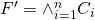where each clause in F contains all negated or non-negated variables, is there an assignment of the variables so that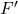is satisfied?

Example: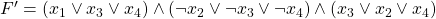the following assignment satisfies: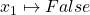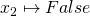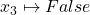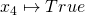The reduction:
In the following reduction we are given an instance of SAT, with the clauses: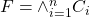. Here each clause is of the form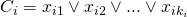and each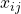is a literal of the form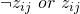Now we build an instance of Monotone SAT from the instance of SAT given above:
For each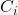we construct two new clauses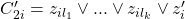and, such that all elements of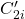are non-negated literals and all terms in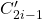are negated literals with the addition of the new special term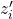. Now let us build a new formula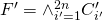this is our instance of Monotone SAT, clauses are either all non-negated or negated.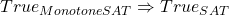:
Notice how we added the extra literalor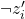to each of the clausesorrespectfully. Now if there is an assignment that satisfies all of the clauses ofthen as only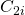or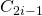may be satisfied by the appended extra literal, one of the clauses must be satisfied by it’s other literals. These literals are also inso such an assignment satisfies all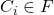.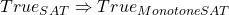:
Using an argument similar to the one above, For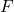to be satisfied there must be at least one literal assignment say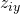that satisfies each clauseNowis in eitheror. This implies that at least one ofor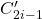is also satisfied by, so simply assign the new termaccordingly to satisfy the clause innot satisfied by(back to me again)

Difficulty: 3.  I like that the reduction involves manipulating the formula, instead of applying logical identities.